22 Apr, 2014

# Simple Equations to Approximate Changes to the Properties of Crude Oil with Changing Temperature

This article describes simple equations to approximate changes to the properties of crude oil with changing temperature.   Changes in crude oil density and specific heat, or heat capacity, can be estimated from graphs and/or more elaborate computer simulation.  The latter generally requires access to a process simulator and characterization data for the crude oil.  A suitable, tuned computer model is likely the most accurate method of estimating the fluid properties, but is not always available.  Direct laboratory measurement is also possible if facilities and oil samples are available and a high degree of accuracy is required.

Graphs, which were originally generated from empirical data, can be useful, and their accuracy is suitable for most engineering applications. However; use of the data in subsequent calculations requires users to interrupt the calculation, look up a number from a graph, and then proceed with the calculation.  The simple curve-fit equations presented provide the required data suitable for use in spreadsheet or hand calculations.

Crude Oil Density

Figure 1A (1) depicts the change in specific gravity with temperature for crude oils of varying API gravity.

This graph was compared with density-temperature data from Table D-1, API Publication 421, “Monographs on Refinery Environmental Control – Management of Water Discharges” (2).  The colored lines in Figure 1B show the API data superimposed on the original graph. The two show good agreement.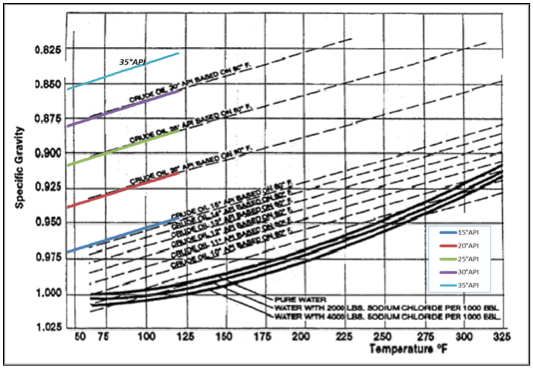Figure 1B Data from API Publication 421 superimposed on Figure 1A (data from (2))

Curve fitting data from Figure 1A resulted in Equation 1 for FPS units.  Equation 2 provides the SI equivalent.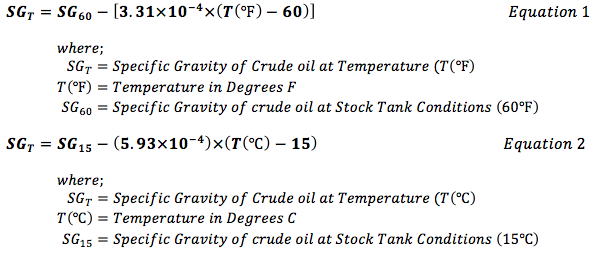Figure 1C displays the output from Equation 1 superimposed as colored lines on the original graph (Figure 1A). Although the simple equation does not align perfectly, results are sufficiently accurate for most engineering calculations.  Compared to the data from API Publication 421,  Equation 1 produces a maximum errors of +0.25% and -0.3%.

Heat Capacity

A similar approach was used to develop a simple equation for the variation of the Heat Capacity or Specific Heat of crude oil as a function of API gravity and Temperature.  Data, extracted from Figure 2A (1), was regressed to obtain the algorithm presented as Equation 3 for FPS units: Equation 4 for SI units.  Note that the algorithm was developed for a crude oil with a UOP index of 11.8 (indicating intermediate, paraffinic-naphthenic crude).  If the UOP index is known, the correction factor illustrated on the graph could be applied to the output from Equation 3 or 4.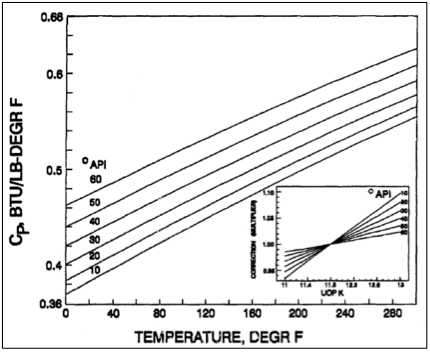Figure 2A Heat capacity of crude oil vs Temperature (1)

The resulting equation is presented as Equation 3 for FPS units, and Equation 4 for SI units.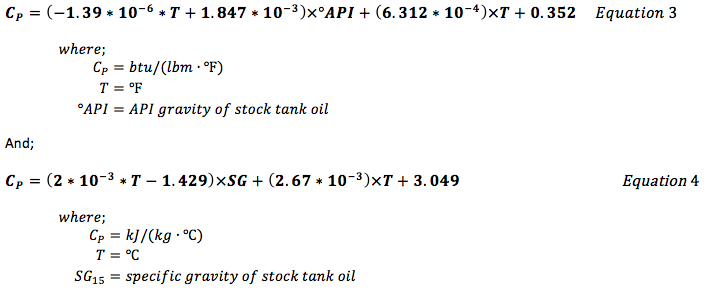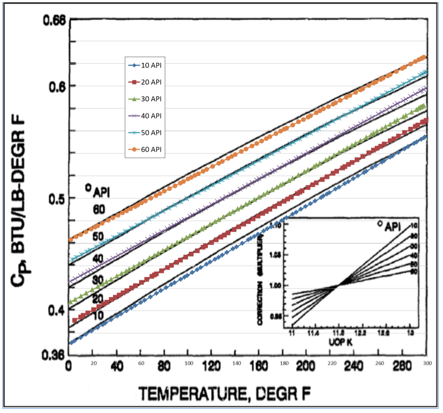Figure 2B Simple Equation 3 superimposed on Figure 2A

Summary

The simple equations provide approximations for the variation of density and specific heat of crude oils of varying API gravity.  Neither algorithm provides a perfect match with the underlying data.  However; data from varying sources do not always correlate.  Figure 3A (1) depicts an alternate source of density correction for crude oils for varying API gravity and temperature.  Figure 3B shows data from API Publication 421 (colored lines) superimposed on a portion of Figure 3A.  Unfortunately, overlap of the data is limited, but clearly there is a very poor correlation – even the trend as API gravity increases is reversed between the API data and the data presented in Figure 3A.  Note that the trend represented in Figure 1A is supported by data from API 421 Appendix D.  These data (from the API Publication 421) are taken here as being most reliable.  Overall, Figure 1A shows quite good agreement with the API data, so the algorithm (Equations 1 and 2) was developed using data from Figure 1A as the source.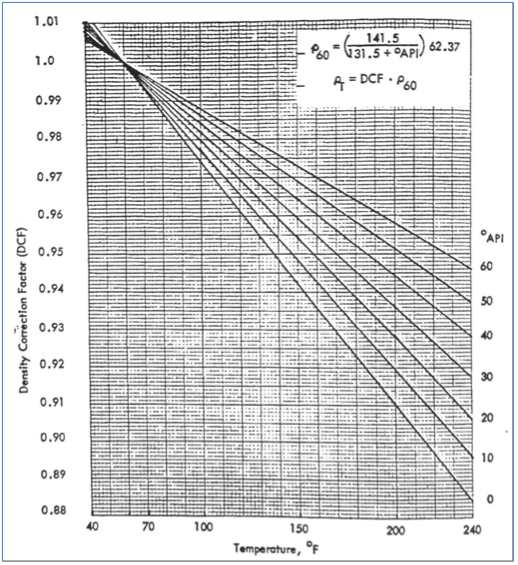Figure 3A Crude Oil Density Correction Factor (Hankinson et al, 1979) (1)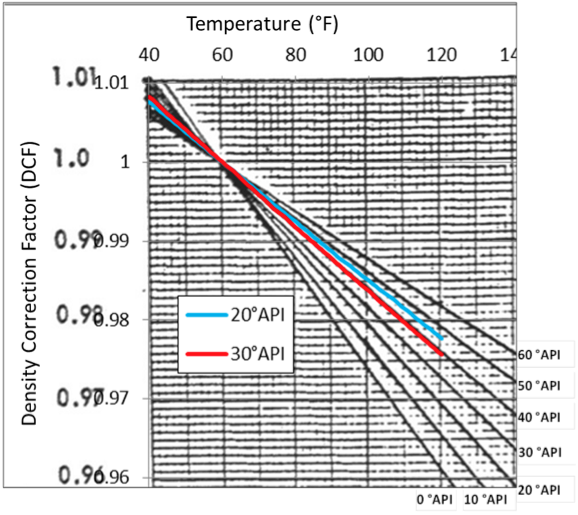Figure 3B Portion of Figure 3 A with data from API 421 superimposed

Works Cited

1. Manning, Francis S. and Thompson, Richard E. OILFIELD PROCESSING Volume Two: Crude Oil. Tulsa : PennWell Publishing Company, 1995. ISBN 0-87814-354-8.

2. Institute, American Petroleum. Management of Water Discharges: Design and Operation of Oil-Water Separators. Washington : API, 1990. API Publication 421.